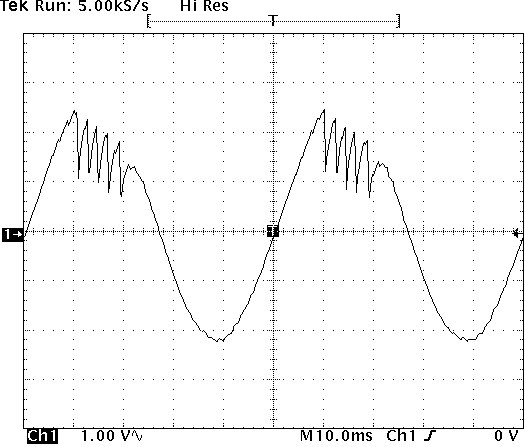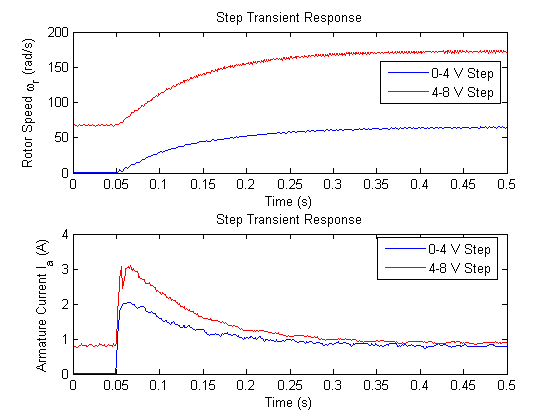# Lab 5b Tips: DC Brush Motor

## Pre-lab

• Do not use Laplace transforms!
• The entire pre-lab should be completed with algebraic manipulation of equations (1-4) and the following trigonometry identity where α = tan-1(b/a).• You can safely assume φ = -α.
• You may verify your expressions numerically using the constant parameters below. Note: J is needed to calculate A and vice versa.
``` ra = 2; % Ohms Laa = 4e-3; % H kt = 0.037; % Nm/A kv = 0.039; % Vs/rad J = 5e-5; % Nms^s/rad Bm = 7e-5; % Nms/rad K1 = 10; % V K2 = 5; % V we = 2*pi*10; % rad/s ```
• Using these parameters, the correct numeric results are

## In-lab

• You can complete the entire lab with this one model.• See Recall Factory Setup
• See AC Coupling Mode
• If you are experiencing amplifier overloading, the supplied current waveform will have fold back. A typical example of current fold back is shown below.• See Average(#) Mode
• Remeber we only measured the friction in motor 1. To account for motor 2, double the measured Bm in the calculations and simulation.
• See Single Trigger Mode. Note: The oscilliscope must be in DC coupling and either sample or hi-res acquisition mode for single trigger mode to work properly. It is easiest to recall factory setup before setting single trigger mode to correct these settings.
• See Paired Cursors
• Alternatively, the following Matlab code may be used to find the time constant of the exponential speed response. Once you have saved data from 'digscope', create an m-file to load the data such as:

``` load oscope_0_4.dat t = oscope_0_4(:,1); % s v = oscope_0_4(:,2); % V wr = oscope_0_4(:,3)/k_vt; % rad/s Ia = oscope_0_4(:,4); % A wr_ss_0_4 = max(wr); % rad/s tm_0_4 = find_tm(t,v,wr); % s ```
Then re-create the 'find_tm' function below in a separate m-file.

``` function delta_t = find_tm(t,v,wr) wr_stop = (max(wr)-min(wr))*(1-exp(-1)) + min(wr); t_start = t(find(v>v(1)+2,1)); t_stop = t(find(wr>wr_stop,1)); delta_t = t_stop-t_start; ```